# Temp_spy

This is an IOT based project. which sends an alert E-mail. it gives an alert message when there is a rise or fall in the temperature.

IntermediateFull instructions provided3 hours206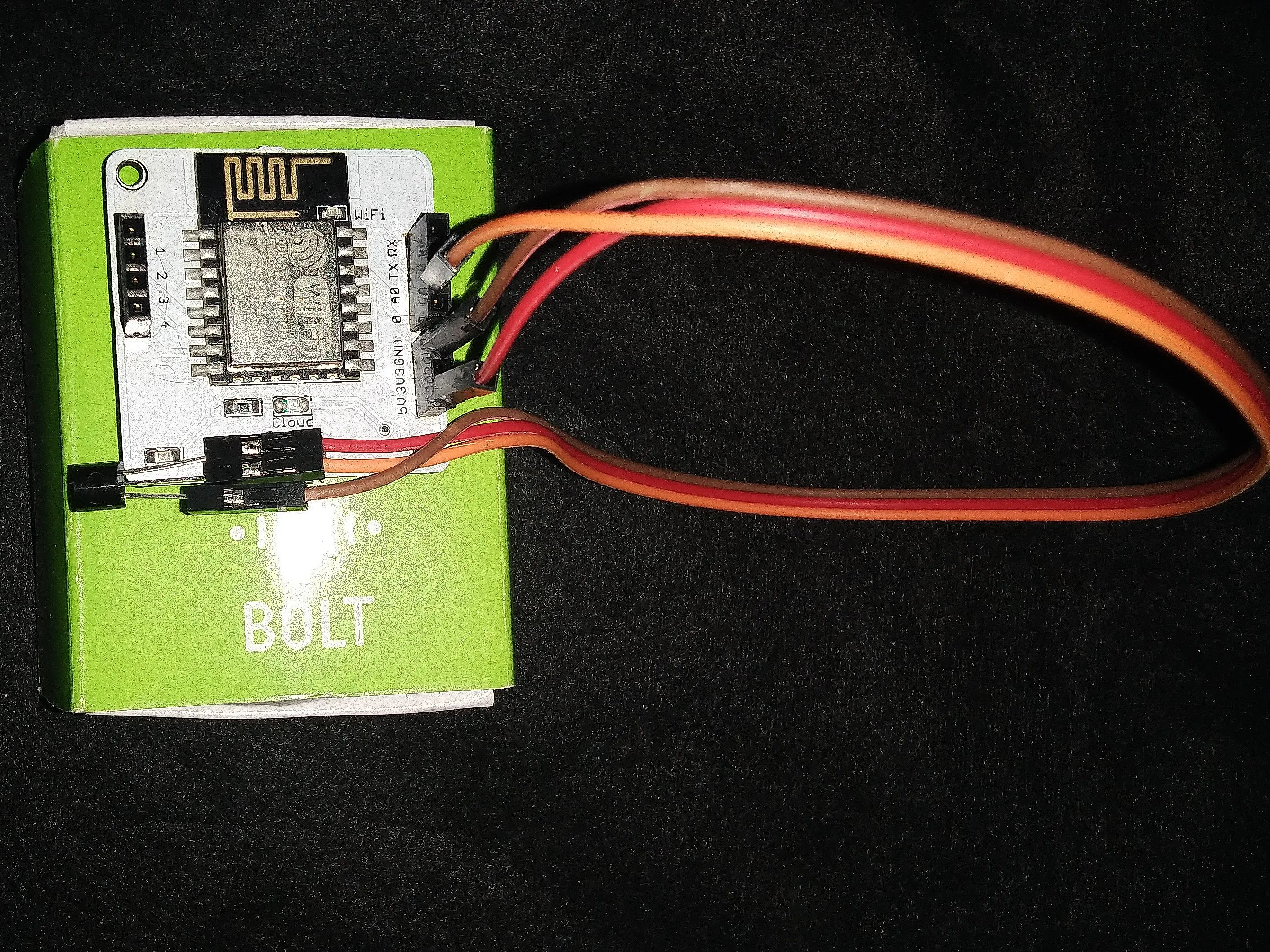## Things used in this project

### Hardware componentsTemperature Sensor
×1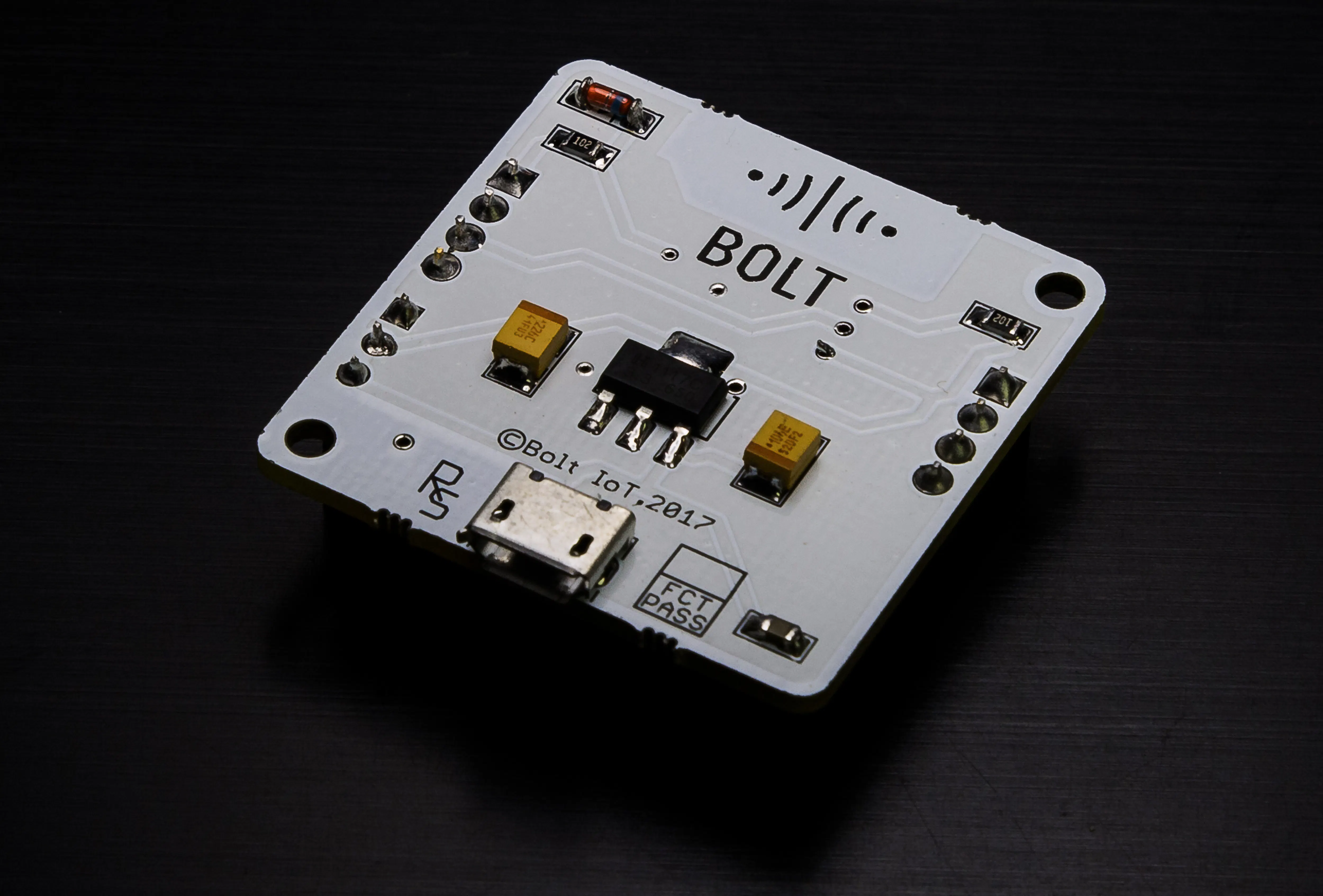Bolt IoT Bolt WiFi Module
×1

### Software apps and online servicesBolt IoT Bolt Cloud

### Hand tools and fabrication machinesPremium Female/Female Jumper Wires, 40 x 3" (75mm)

## Custom parts and enclosures

hardware and components for tem_spy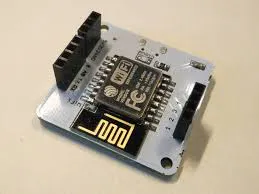## Schematics

### circuit connection

circuit connection for temp_spy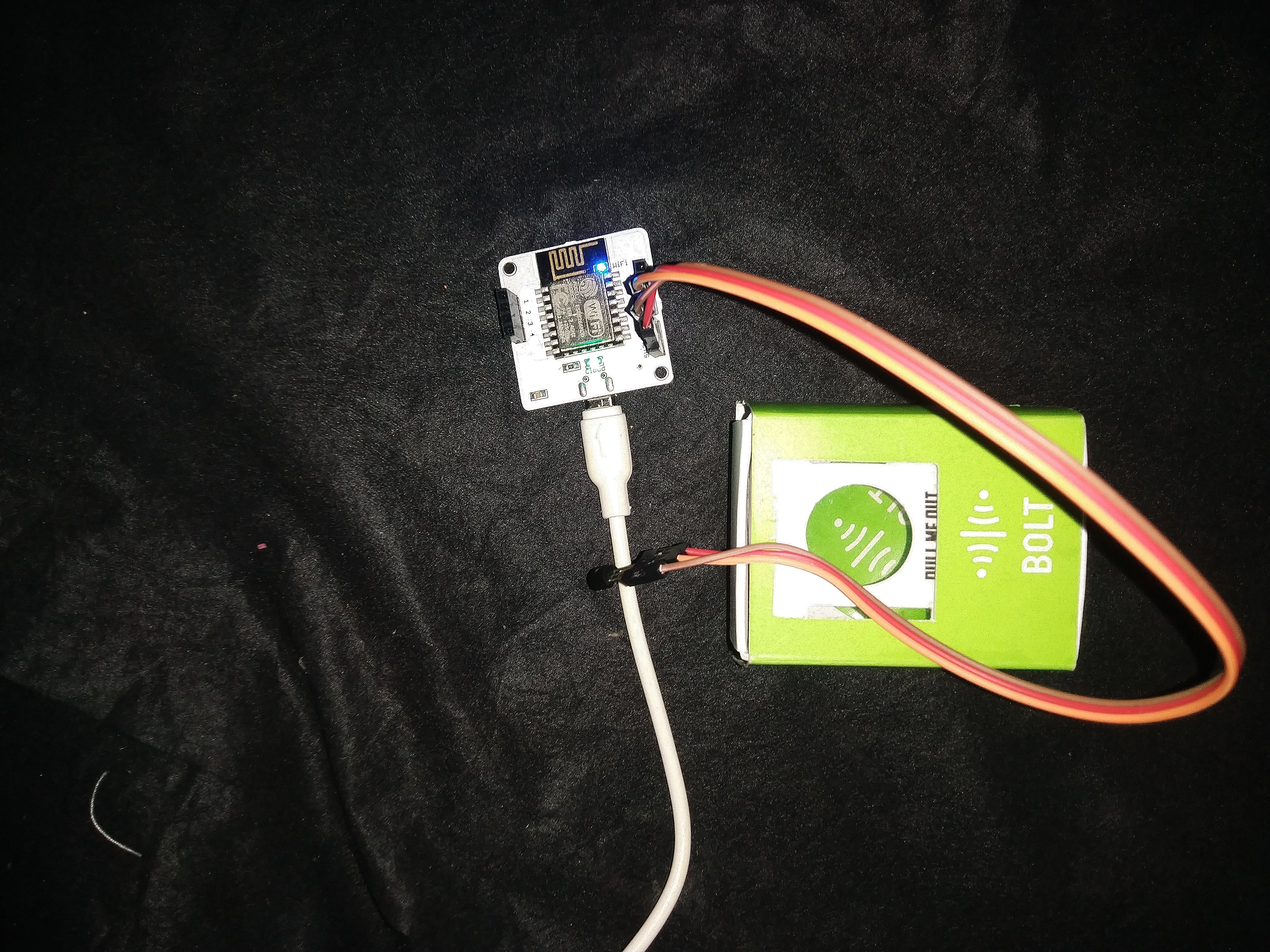### circuit connection for temp_spy

this is the hardware setup and connection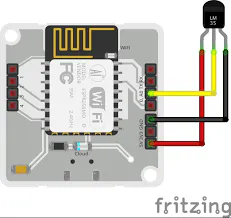## Code

### code for temp_spy

Python
This is the source code for Temp_spy where it records the temperature and if there is a sudden rise or fall in the temperature it send an alert Email.
```import main,json,time,math,statistics
from boltiot import Email,Bolt
def comp_bound(hdata,frame_size,factor):
if len(hdata)<frame_size:
return None

if len(hdata)>frame_size:
del hdata[0:len(hdata)-frame_size]
mn = statistics.mean(hdata)
variance = 0
for data in hdata:
variance += math.pow((data-mn),2)
## to compute z score
zn = factor *math.sqrt(variance/frame_size)
## to compute bounds
h_bound = hdata[frame_size-1]+zn
l_bound = hdata[frame_size-1]-zn
return [h_bound,l_bound]

mybolt = Bolt(main.api_key,main.device_id)
hdata = []
max = 240
min = 190
while True:
if data['success']!= 1:
print("there was an error while retriving the data")
print("this is the error:"+data['value'])
time.sleep(10)
continue

print("this the value"+data['value'])
sensor_value = 0
try:
sensor_value = int(data['value'])
except e:
print("the was an error while parsing the response:",e)
continue

bound = comp_bound(hdata,main.Frame_size,main.mul_fact)
if not bound:
required_data = main.Frame_size - len(hdata)
print("need more data for computation",required_data,"more data")
hdata.append(int(data['value']))
time.sleep(10)
continue
try:
if sensor_value > bound or sensor_value > 240:
print("the temperature increased suddenly. Sending an email")
response = mailer.send_email("alert email","Some one opened the refrigerator")
print("this is the response" +str(response_text['message']))
elif sensor_value < bound and sensor_value < 190:
print("the light level decreased suddenly.Sending an email")
response = mailer.send_email("alert mail","Some one closed the refrigerator")
print("this is the response"+str(response_text['message']))
hdata.append(sensor_value);
except Exception as e:
print("error")
time.sleep(10)
```

## Credits

### malolan aravamuthan

1 project • 0 followers